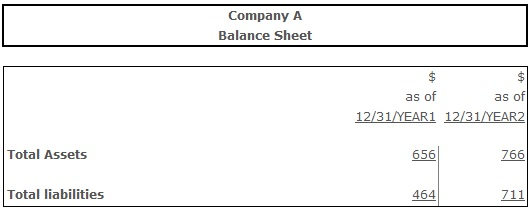# Debt Ratio

Debt Ratio – a ratio indicating firm’s long-term debt-paying ability through comparing its liabilities to total assets. It can be calculated by dividing the company's total liabilities (current and noncurrent) by its total assets.

Normative values for the debt ratio range from 0,4 to 0,6. But they can vary depending on the industry. If the firm's debt ratio is higher than competitors', this can lead to the price increase of the financial resources attraction.

The high value of the debt ratio itself does not necessarily indicate a high level of bankruptcy risk for a company in the near future. For example, in the situation when 80% of company's sources of finance is debt, but it is able to generate consistent positive net cash flow - it shouldn't face any problems to meet its credit obligations. Different business cycles, consistent technologies development, active competitors' actions, changes in consumer taste - this all leads to the changes of the market situation. Facing the decrease of sales volume may cause the change of the net cash flow from positive to negative. In such conditions the high level of debt would lead to the fast bankruptcy of a company, while firms with a good level of the financial sustainability and enough ownership equity would be able to continue functioning. Assuming this, higher than normative debt ratio value doesn't indicate the upcoming in the near future bankruptcy of a company, but means high enough level of risk in a long-term perspective.

Contrary to the above-described situation, low level of the debt ratio can witness the incomplete usage of company's potential. This can be stated because credit capital allows to increase sales volume, intensify the financial, operating or investing activity. The low share of credit capital is a negative thing if the financial leverage effect (an indicator measuring the increase of profitability of the equity with the involvement of an additional dollar of debt) is positive.

## Resolving the problems with the debt ratio exceeding the normative range:

If the debt ratio is lower than normative, it is necessary to seek for the additional debt funds attraction. However, this action is only reasonable if the return on investment or return on equity increase is expected. In other words, if every involved dollar of debt will be able to generate the financial result greater than the cost of debt, this action would be reasonable. In case the debt ratio is higher than normative the company can take following measures:

• apply changes to the current dividend policy and reinvest received profit into the company's everyday operations;
• involve additional funds of current owners or new investors;
• optimize the current structure of assets to decrease the necessity of new sources of finance, etc.

## Formula(s):

Debt Ratio = Total Liabilities ÷ Total Assets

Debt Ratio = (Total Assets - Total Equity) ÷ Total Assets

## Example:Debt Ratio (Year 1) = 464 ÷ 656 = 0,70

Debt Ratio (Year 2) = 711 ÷ 766 = 0,92

Debt ratio in year 1 was 0,7, meaning that each \$1 of assets was financed by \$0,7 of debt, and this value lies within the “distress” zone (higher than 0,6). In year 2 the debt ratio was 0,92, indicating the critical increase of the financial and credit risk comparing to year 1.

## Conclusion:

Debt ratio reflects the share of assets financed by liabilities. Optimal is the situation, when the debt ratio lies within the normative range from 0,4 to 0,6.